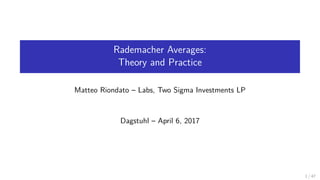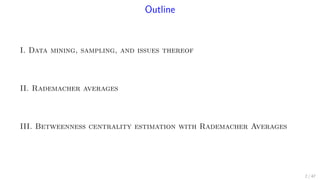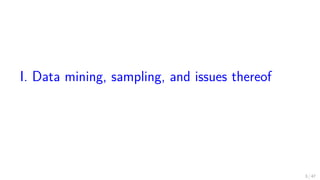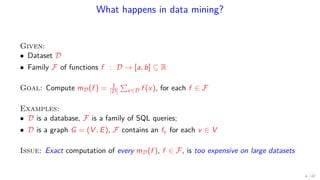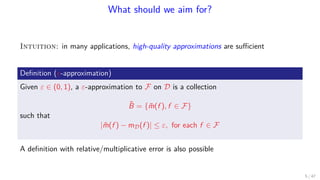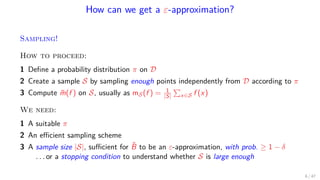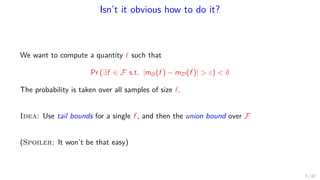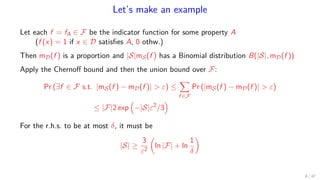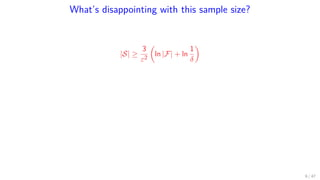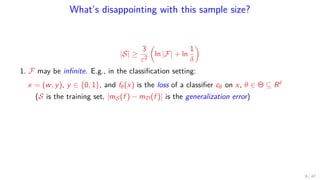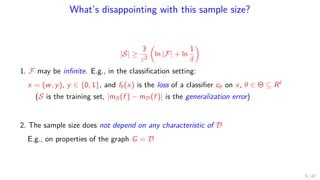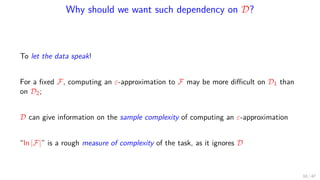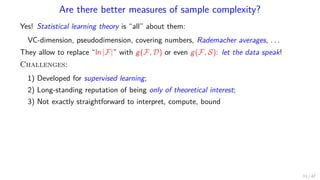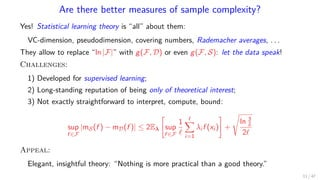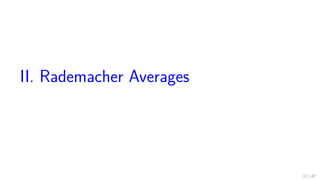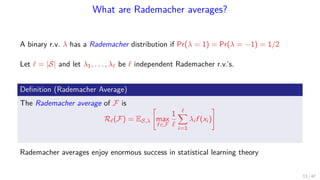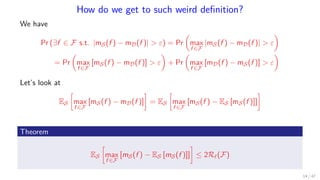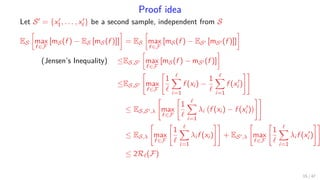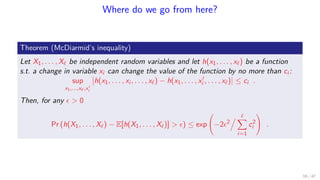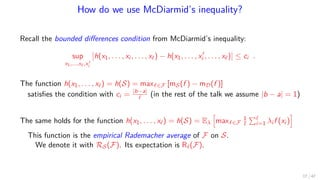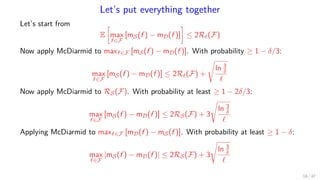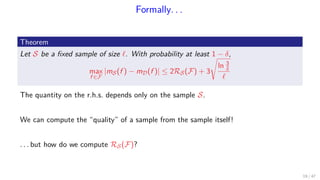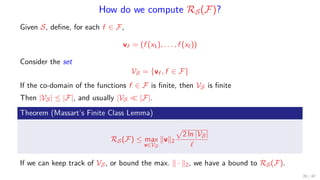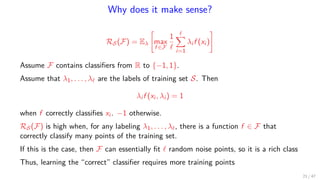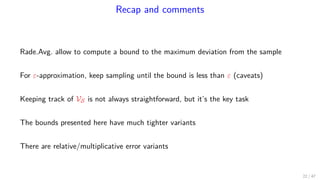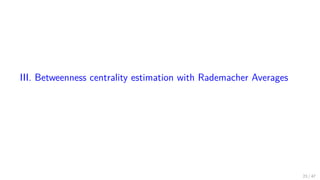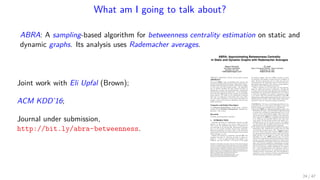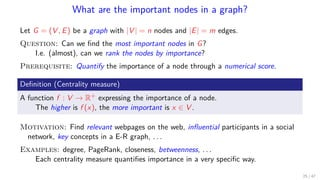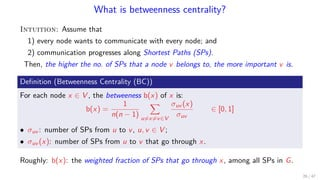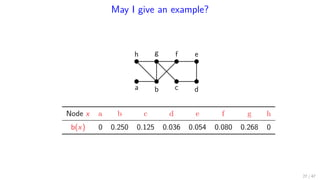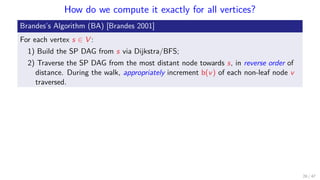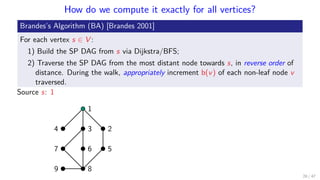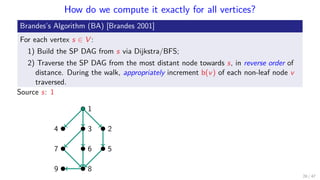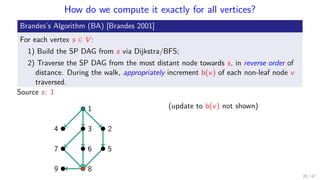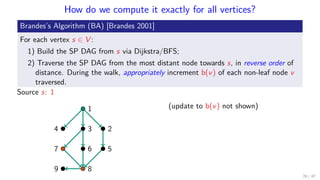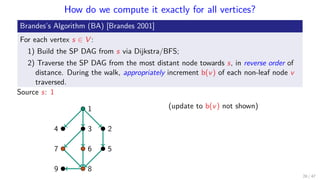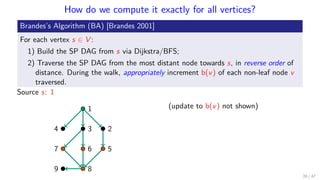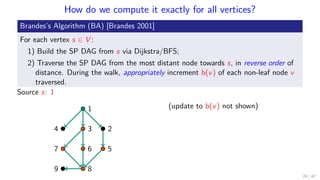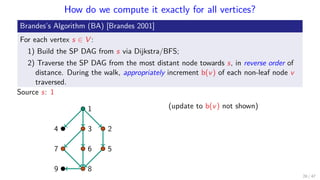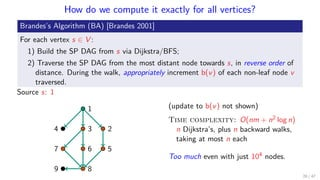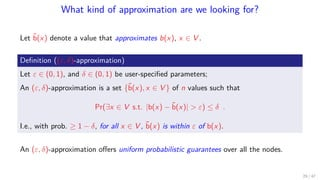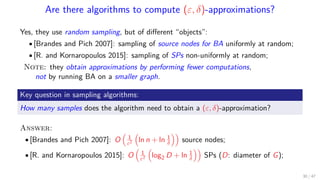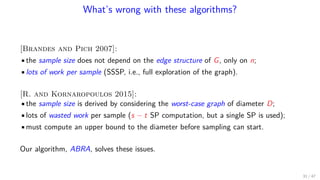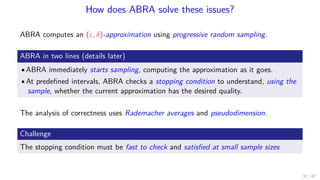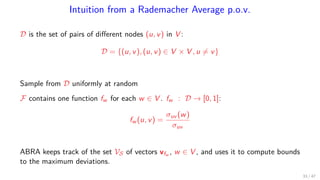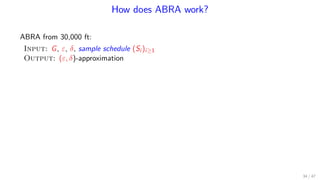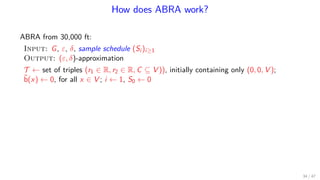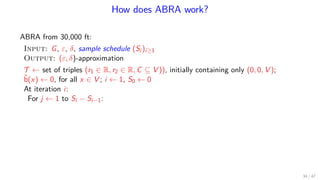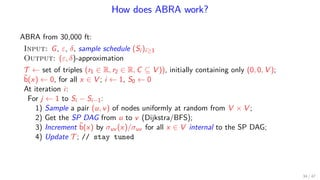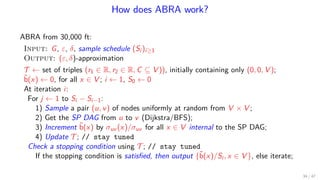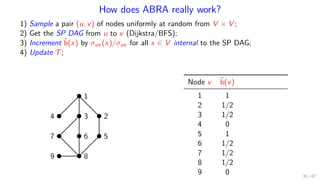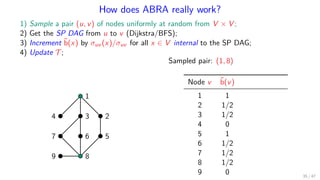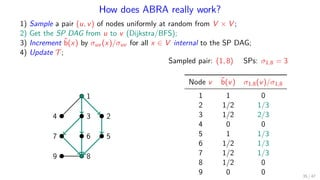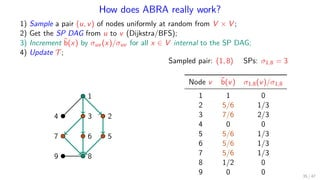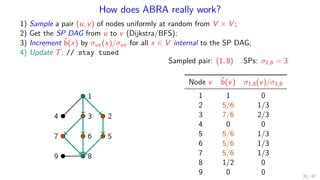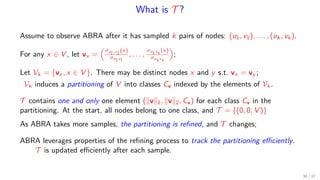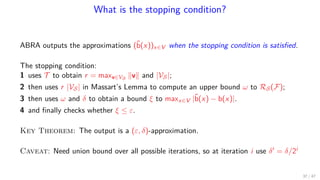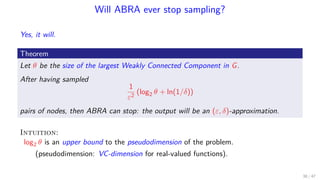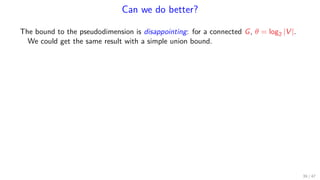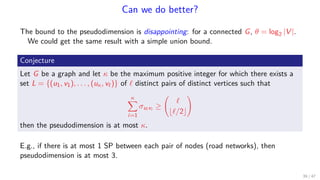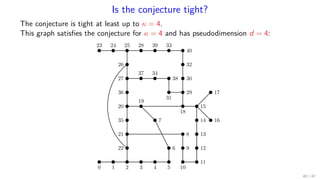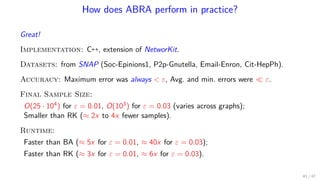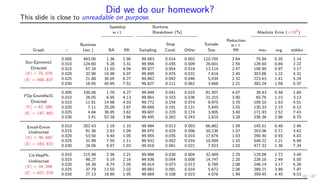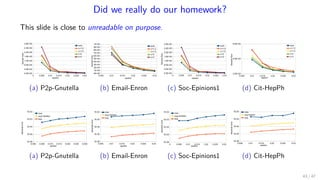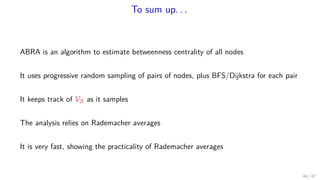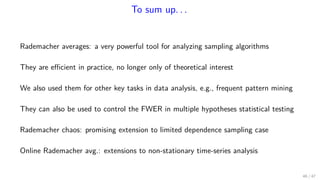1 of 69

### Rademacher Averages: Theory and Practice

1. Rademacher Averages: Theory and Practice Matteo Riondato – Labs, Two Sigma Investments LP Dagstuhl – April 6, 2017 1 / 47
2. Outline I. Data mining, sampling, and issues thereof II. Rademacher averages III. Betweenness centrality estimation with Rademacher Averages 2 / 47
3. I. Data mining, sampling, and issues thereof 3 / 47
4. What happens in data mining? Given: • Dataset D • Family F of functions f : D → [a, b] ⊆ R Goal: Compute mD(f ) = 1 |D| x∈D f (x), for each f ∈ F Examples: • D is a database, F is a family of SQL queries; • D is a graph G = (V , E), F contains an fv for each v ∈ V Issue: Exact computation of every mD(f ), f ∈ F, is too expensive on large datasets 4 / 47
5. What should we aim for? Intuition: in many applications, high-quality approximations are suﬃcient Deﬁnition (ε-approximation) Given ε ∈ (0, 1), a ε-approximation to F on D is a collection ˜B = { ˜m(f ), f ∈ F} such that | ˜m(f ) − mD(f )| ≤ ε, for each f ∈ F A deﬁnition with relative/multiplicative error is also possible 5 / 47
6. How can we get a ε-approximation? Sampling! How to proceed: 1 Deﬁne a probability distribution π on D 2 Create a sample S by sampling enough points independently from D according to π 3 Compute ˜m(f ) on S, usually as mS(f ) = 1 |S| x∈S f (x) We need: 1 A suitable π 2 An eﬃcient sampling scheme 3 A sample size |S|, suﬃcient for ˜B to be an ε-approximation, with prob. ≥ 1 − δ . . . or a stopping condition to understand whether S is large enough 6 / 47
7. Isn’t it obvious how to do it? We want to compute a quantity such that Pr (∃f ∈ F s.t. |mS(f ) − mD(f )| > ε) < δ The probability is taken over all samples of size . Idea: Use tail bounds for a single f , and then the union bound over F (Spoiler: It won’t be that easy) 7 / 47
8. Let’s make an example Let each f = fA ∈ F be the indicator function for some property A (f (x) = 1 if x ∈ D satisﬁes A, 0 othw.) Then mD(f ) is a proportion and |S|mS(f ) has a Binomial distribution B(|S|, mD(f )) Apply the Chernoﬀ bound and then the union bound over F: Pr (∃f ∈ F s.t. |mS(f ) − mD(f )| > ε) ≤ f ∈F Pr (|mS(f ) − mD(f )| > ε) ≤ |F|2 exp −|S|ε2 /3 For the r.h.s. to be at most δ, it must be |S| ≥ 3 ε2 ln |F| + ln 1 δ 8 / 47
9. What’s disappointing with this sample size? |S| ≥ 3 ε2 ln |F| + ln 1 δ 9 / 47
10. What’s disappointing with this sample size? |S| ≥ 3 ε2 ln |F| + ln 1 δ 1. F may be inﬁnite. E.g., in the classiﬁcation setting: x = (w, y), y ∈ {0, 1}, and fθ(x) is the loss of a classiﬁer cθ on x, θ ∈ Θ ⊆ R (S is the training set, |mS(f ) − mD(f )| is the generalization error) 9 / 47
11. What’s disappointing with this sample size? |S| ≥ 3 ε2 ln |F| + ln 1 δ 1. F may be inﬁnite. E.g., in the classiﬁcation setting: x = (w, y), y ∈ {0, 1}, and fθ(x) is the loss of a classiﬁer cθ on x, θ ∈ Θ ⊆ R (S is the training set, |mS(f ) − mD(f )| is the generalization error) 2. The sample size does not depend on any characteristic of D E.g., on properties of the graph G = D 9 / 47
12. Why should we want such dependency on D? To let the data speak! For a ﬁxed F, computing an ε-approximation to F may be more diﬃcult on D1 than on D2; D can give information on the sample complexity of computing an ε-approximation “ln |F|” is a rough measure of complexity of the task, as it ignores D 10 / 47
13. Are there better measures of sample complexity? Yes! Statistical learning theory is “all” about them: VC-dimension, pseudodimension, covering numbers, Rademacher averages, . . . They allow to replace “ln |F|” with g(F, D) or even g(F, S): let the data speak! Challenges: 1) Developed for supervised learning; 2) Long-standing reputation of being only of theoretical interest; 3) Not exactly straightforward to interpret, compute, bound 11 / 47
14. Are there better measures of sample complexity? Yes! Statistical learning theory is “all” about them: VC-dimension, pseudodimension, covering numbers, Rademacher averages, . . . They allow to replace “ln |F|” with g(F, D) or even g(F, S): let the data speak! Challenges: 1) Developed for supervised learning; 2) Long-standing reputation of being only of theoretical interest; 3) Not exactly straightforward to interpret, compute, bound: sup f ∈F |mS(f ) − mD(f )| ≤ 2Eλ sup f ∈F 1 i=1 λi f (xi ) + ln 3 δ 2 Appeal: Elegant, insightful theory: “Nothing is more practical than a good theory.” 11 / 47
15. II. Rademacher Averages 12 / 47
16. What are Rademacher averages? A binary r.v. λ has a Rademacher distribution if Pr(λ = 1) = Pr(λ = −1) = 1/2 Let = |S| and let λ1, . . . , λ be independent Rademacher r.v.’s. Deﬁnition (Rademacher Average) The Rademacher average of F is R (F) = ES,λ max f ∈F 1 i=1 λi f (xi ) Rademacher averages enjoy enormous success in statistical learning theory 13 / 47
17. How do we get to such weird deﬁnition? We have Pr (∃f ∈ F s.t. |mS(f ) − mD(f )| > ε) = Pr max f ∈F |mS(f ) − mD(f )| > ε = Pr max f ∈F [mS(f ) − mD(f )] > ε + Pr max f ∈F [mD(f ) − mS(f )] > ε Let’s look at ES max f ∈F [mS(f ) − mD(f )] = ES max f ∈F [mS(f ) − ES [mS(f )]] Theorem ES max f ∈F [mS(f ) − ES [mS(f )]] ≤ 2R (F) 14 / 47
18. Proof idea Let S = {x1, . . . , x } be a second sample, independent from S ES max f ∈F [mS(f ) − ES [mS(f )]] = ES max f ∈F [mS(f ) − ES [mS (f )]] (Jensen’s Inequality) ≤ES,S max f ∈F [mS(f ) − mS (f )] ≤ES,S max f ∈F 1 i=1 f (xi ) − 1 i=1 f (xi ) ≤ ES,S ,λ max f ∈F 1 i=1 λi f (xi ) − f (xi ) ≤ ES,λ max f ∈F 1 i=1 λi f (xi ) + ES ,λ max f ∈F 1 i=1 λi f (xi ) ≤ 2R (F) 15 / 47
19. Where do we go from here? Theorem (McDiarmid’s inequality) Let X1, . . . , X be independent random variables and let h(x1, . . . , x ) be a function s.t. a change in variable xi can change the value of the function by no more than ci : sup x1,...,x ,xi h(x1, . . . , xi , . . . , x ) − h(x1, . . . , xi , . . . , x ) ≤ ci . Then, for any > 0 Pr (h(X1, . . . , X ) − E[h(X1, . . . , X )] > ) ≤ exp −2 2 i=1 c2 i . 16 / 47
20. How do we use McDiarmid’s inequality? Recall the bounded diﬀerences condition from McDiarmid’s inequality: sup x1,...,x ,xi h(x1, . . . , xi , . . . , x ) − h(x1, . . . , xi , . . . , x ) ≤ ci . The function h(x1, . . . , x ) = h(S) = maxf ∈F [mS(f ) − mD(f )] satisﬁes the condition with ci = |b−a| (in the rest of the talk we assume |b − a| = 1) The same holds for the function h(x1, . . . , x ) = h(S) = Eλ maxf ∈F 1 i=1 λi f (xi ) This function is the empirical Rademacher average of F on S. We denote it with RS(F). Its expectation is R (F). 17 / 47
21. Let’s put everything together Let’s start from E max f ∈F [mS(f ) − mD(f )] ≤ 2R (F) Now apply McDiarmid to maxf ∈F [mS(f ) − mD(f )]. With probability ≥ 1 − δ/3: max f ∈F [mS(f ) − mD(f )] ≤ 2R (F) + ln 3 δ Now apply McDiarmid to RS(F). With probability at least ≥ 1 − 2δ/3: max f ∈F [mS(f ) − mD(f )] ≤ 2RS(F) + 3 ln 3 δ Applying McDiarmid to maxf ∈F [mD(f ) − mS(f )]. With probability at least ≥ 1 − δ: max f ∈F |mS(f ) − mD(f )| ≤ 2RS(F) + 3 ln 3 δ 18 / 47
22. Formally. . . Theorem Let S be a ﬁxed sample of size . With probability at least 1 − δ, max f ∈F |mS(f ) − mD(f )| ≤ 2RS(F) + 3 ln 3 δ The quantity on the r.h.s. depends only on the sample S. We can compute the “quality” of a sample from the sample itself! . . . but how do we compute RS(F)? 19 / 47
23. How do we compute RS(F)? Given S, deﬁne, for each f ∈ F, vf = (f (x1), . . . , f (x )) Consider the set VS = {vf , f ∈ F} If the co-domain of the functions f ∈ F is ﬁnite, then VS is ﬁnite Then |VS| ≤ |F|, and usually |VS |F|. Theorem (Massart’s Finite Class Lemma) RS(F) ≤ max v∈VS v 2 2 ln |VS| If we can keep track of VS, or bound the max. · 2, we have a bound to RS(F). 20 / 47
24. Why does it make sense? RS(F) = Eλ max f ∈F 1 i=1 λi f (xi ) Assume F contains classiﬁers from R to {−1, 1}. Assume that λ1, . . . , λ are the labels of training set S. Then λi f (xi , λi ) = 1 when f correctly classiﬁes xi . −1 otherwise. RS(F) is high when, for any labeling λ1, . . . , λ , there is a function f ∈ F that correctly classify many points of the training set. If this is the case, then F can essentially ﬁt random noise points, so it is a rich class Thus, learning the “correct” classiﬁer requires more training points 21 / 47
25. Recap and comments Rade.Avg. allow to compute a bound to the maximum deviation from the sample For ε-approximation, keep sampling until the bound is less than ε (caveats) Keeping track of VS is not always straightforward, but it’s the key task The bounds presented here have much tighter variants There are relative/multiplicative error variants 22 / 47
26. III. Betweenness centrality estimation with Rademacher Averages 23 / 47
28. What are the important nodes in a graph? Let G = (V , E) be a graph with |V | = n nodes and |E| = m edges. Question: Can we ﬁnd the most important nodes in G? I.e. (almost), can we rank the nodes by importance? Prerequisite: Quantify the importance of a node through a numerical score. Deﬁnition (Centrality measure) A function f : V → R+ expressing the importance of a node. The higher is f (x), the more important is x ∈ V . Motivation: Find relevant webpages on the web, inﬂuential participants in a social network, key concepts in a E-R graph, . . . Examples: degree, PageRank, closeness, betweenness, . . . Each centrality measure quantiﬁes importance in a very speciﬁc way. 25 / 47
29. What is betweenness centrality? Intuition: Assume that 1) every node wants to communicate with every node; and 2) communication progresses along Shortest Paths (SPs). Then, the higher the no. of SPs that a node v belongs to, the more important v is. Deﬁnition (Betweenness Centrality (BC)) For each node x ∈ V , the betweeness b(x) of x is: b(x) = 1 n(n − 1) u=x=v∈V σuv (x) σuv ∈ [0, 1] • σuv : number of SPs from u to v, u, v ∈ V ; • σuv (x): number of SPs from u to v that go through x. Roughly: b(x): the weighted fraction of SPs that go through x, among all SPs in G. 26 / 47
30. May I give an example? a h b g f e c d Node x a b c d e f g h b(x) 0 0.250 0.125 0.036 0.054 0.080 0.268 0 27 / 47
31. How do we compute it exactly for all vertices? Brandes’s Algorithm (BA) [Brandes 2001] For each vertex s ∈ V : 1) Build the SP DAG from s via Dijkstra/BFS; 2) Traverse the SP DAG from the most distant node towards s, in reverse order of distance. During the walk, appropriately increment b(v) of each non-leaf node v traversed. 28 / 47
32. How do we compute it exactly for all vertices? Brandes’s Algorithm (BA) [Brandes 2001] For each vertex s ∈ V : 1) Build the SP DAG from s via Dijkstra/BFS; 2) Traverse the SP DAG from the most distant node towards s, in reverse order of distance. During the walk, appropriately increment b(v) of each non-leaf node v traversed. Source s: 1 1 234 567 89 28 / 47
33. How do we compute it exactly for all vertices? Brandes’s Algorithm (BA) [Brandes 2001] For each vertex s ∈ V : 1) Build the SP DAG from s via Dijkstra/BFS; 2) Traverse the SP DAG from the most distant node towards s, in reverse order of distance. During the walk, appropriately increment b(v) of each non-leaf node v traversed. Source s: 1 1 234 567 89 28 / 47
34. How do we compute it exactly for all vertices? Brandes’s Algorithm (BA) [Brandes 2001] For each vertex s ∈ V : 1) Build the SP DAG from s via Dijkstra/BFS; 2) Traverse the SP DAG from the most distant node towards s, in reverse order of distance. During the walk, appropriately increment b(v) of each non-leaf node v traversed. Source s: 1 1 234 567 89 (update to b(v) not shown) 28 / 47
35. How do we compute it exactly for all vertices? Brandes’s Algorithm (BA) [Brandes 2001] For each vertex s ∈ V : 1) Build the SP DAG from s via Dijkstra/BFS; 2) Traverse the SP DAG from the most distant node towards s, in reverse order of distance. During the walk, appropriately increment b(v) of each non-leaf node v traversed. Source s: 1 1 234 567 89 (update to b(v) not shown) 28 / 47
36. How do we compute it exactly for all vertices? Brandes’s Algorithm (BA) [Brandes 2001] For each vertex s ∈ V : 1) Build the SP DAG from s via Dijkstra/BFS; 2) Traverse the SP DAG from the most distant node towards s, in reverse order of distance. During the walk, appropriately increment b(v) of each non-leaf node v traversed. Source s: 1 1 234 567 89 (update to b(v) not shown) 28 / 47
37. How do we compute it exactly for all vertices? Brandes’s Algorithm (BA) [Brandes 2001] For each vertex s ∈ V : 1) Build the SP DAG from s via Dijkstra/BFS; 2) Traverse the SP DAG from the most distant node towards s, in reverse order of distance. During the walk, appropriately increment b(v) of each non-leaf node v traversed. Source s: 1 1 234 567 89 (update to b(v) not shown) 28 / 47
38. How do we compute it exactly for all vertices? Brandes’s Algorithm (BA) [Brandes 2001] For each vertex s ∈ V : 1) Build the SP DAG from s via Dijkstra/BFS; 2) Traverse the SP DAG from the most distant node towards s, in reverse order of distance. During the walk, appropriately increment b(v) of each non-leaf node v traversed. Source s: 1 1 234 567 89 (update to b(v) not shown) 28 / 47
39. How do we compute it exactly for all vertices? Brandes’s Algorithm (BA) [Brandes 2001] For each vertex s ∈ V : 1) Build the SP DAG from s via Dijkstra/BFS; 2) Traverse the SP DAG from the most distant node towards s, in reverse order of distance. During the walk, appropriately increment b(v) of each non-leaf node v traversed. Source s: 1 1 234 567 89 (update to b(v) not shown) 28 / 47
40. How do we compute it exactly for all vertices? Brandes’s Algorithm (BA) [Brandes 2001] For each vertex s ∈ V : 1) Build the SP DAG from s via Dijkstra/BFS; 2) Traverse the SP DAG from the most distant node towards s, in reverse order of distance. During the walk, appropriately increment b(v) of each non-leaf node v traversed. Source s: 1 1 234 567 89 (update to b(v) not shown) Time complexity: O(nm + n2 log n) n Dijkstra’s, plus n backward walks, taking at most n each Too much even with just 104 nodes. 28 / 47
41. What kind of approximation are we looking for? Let ˜b(x) denote a value that approximates b(x), x ∈ V . Deﬁnition ((ε, δ)-approximation) Let ε ∈ (0, 1), and δ ∈ (0, 1) be user-speciﬁed parameters; An (ε, δ)-approximation is a set {˜b(x), x ∈ V } of n values such that Pr(∃x ∈ V s.t. |b(x) − ˜b(x)| > ε) ≤ δ . I.e., with prob. ≥ 1 − δ, for all x ∈ V , ˜b(x) is within ε of b(x). An (ε, δ)-approximation oﬀers uniform probabilistic guarantees over all the nodes. 29 / 47
42. Are there algorithms to compute (ε, δ)-approximations? Yes, they use random sampling, but of diﬀerent “objects”: •[Brandes and Pich 2007]: sampling of source nodes for BA uniformly at random; •[R. and Kornaropoulos 2015]: sampling of SPs non-uniformly at random; Note: they obtain approximations by performing fewer computations, not by running BA on a smaller graph. Key question in sampling algorithms: How many samples does the algorithm need to obtain a (ε, δ)-approximation? Answer: •[Brandes and Pich 2007]: O 1 ε2 ln n + ln 1 δ source nodes; •[R. and Kornaropoulos 2015]: O 1 ε2 log2 D + ln 1 δ SPs (D: diameter of G); 30 / 47
43. What’s wrong with these algorithms? [Brandes and Pich 2007]: •the sample size does not depend on the edge structure of G, only on n; •lots of work per sample (SSSP, i.e., full exploration of the graph). [R. and Kornaropoulos 2015]: •the sample size is derived by considering the worst-case graph of diameter D; •lots of wasted work per sample (s − t SP computation, but a single SP is used); •must compute an upper bound to the diameter before sampling can start. Our algorithm, ABRA, solves these issues. 31 / 47
44. How does ABRA solve these issues? ABRA computes an (ε, δ)-approximation using progressive random sampling. ABRA in two lines (details later) •ABRA immediately starts sampling, computing the approximation as it goes. •At predeﬁned intervals, ABRA checks a stopping condition to understand, using the sample, whether the current approximation has the desired quality. The analysis of correctness uses Rademacher averages and pseudodimension. Challenge The stopping condition must be fast to check and satisﬁed at small sample sizes 32 / 47
45. Intuition from a Rademacher Average p.o.v. D is the set of pairs of diﬀerent nodes (u, v) in V : D = {(u, v), (u, v) ∈ V × V , u = v} Sample from D uniformly at random F contains one function fw for each w ∈ V . fw : D → [0, 1]: fw (u, v) = σuv (w) σuv ABRA keeps track of the set VS of vectors vfw , w ∈ V , and uses it to compute bounds to the maximum deviations. 33 / 47
46. How does ABRA work? ABRA from 30,000 ft: Input: G, ε, δ, sample schedule (Si )i≥1 Output: (ε, δ)-approximation 34 / 47
47. How does ABRA work? ABRA from 30,000 ft: Input: G, ε, δ, sample schedule (Si )i≥1 Output: (ε, δ)-approximation T ← set of triples (r1 ∈ R, r2 ∈ R, C ⊆ V )), initially containing only (0, 0, V ); ˜b(x) ← 0, for all x ∈ V ; i ← 1, S0 ← 0 34 / 47
48. How does ABRA work? ABRA from 30,000 ft: Input: G, ε, δ, sample schedule (Si )i≥1 Output: (ε, δ)-approximation T ← set of triples (r1 ∈ R, r2 ∈ R, C ⊆ V )), initially containing only (0, 0, V ); ˜b(x) ← 0, for all x ∈ V ; i ← 1, S0 ← 0 At iteration i: For j ← 1 to Si − Si−1: 34 / 47
49. How does ABRA work? ABRA from 30,000 ft: Input: G, ε, δ, sample schedule (Si )i≥1 Output: (ε, δ)-approximation T ← set of triples (r1 ∈ R, r2 ∈ R, C ⊆ V )), initially containing only (0, 0, V ); ˜b(x) ← 0, for all x ∈ V ; i ← 1, S0 ← 0 At iteration i: For j ← 1 to Si − Si−1: 1) Sample a pair (u, v) of nodes uniformly at random from V × V ; 2) Get the SP DAG from u to v (Dijkstra/BFS); 3) Increment ˜b(x) by σuv (x)/σuv for all x ∈ V internal to the SP DAG; 4) Update T ; // stay tuned 34 / 47
50. How does ABRA work? ABRA from 30,000 ft: Input: G, ε, δ, sample schedule (Si )i≥1 Output: (ε, δ)-approximation T ← set of triples (r1 ∈ R, r2 ∈ R, C ⊆ V )), initially containing only (0, 0, V ); ˜b(x) ← 0, for all x ∈ V ; i ← 1, S0 ← 0 At iteration i: For j ← 1 to Si − Si−1: 1) Sample a pair (u, v) of nodes uniformly at random from V × V ; 2) Get the SP DAG from u to v (Dijkstra/BFS); 3) Increment ˜b(x) by σuv (x)/σuv for all x ∈ V internal to the SP DAG; 4) Update T ; // stay tuned Check a stopping condition using T ; // stay tuned If the stopping condition is satisﬁed, then output {˜b(x)/Si , x ∈ V }, else iterate; 34 / 47
51. How does ABRA really work? 1) Sample a pair (u, v) of nodes uniformly at random from V × V ; 2) Get the SP DAG from u to v (Dijkstra/BFS); 3) Increment ˜b(x) by σuv (x)/σuv for all x ∈ V internal to the SP DAG; 4) Update T ; 1 234 567 89 Node v ˜b(v) 1 1 2 1/2 3 1/2 4 0 5 1 6 1/2 7 1/2 8 1/2 9 0 35 / 47
52. How does ABRA really work? 1) Sample a pair (u, v) of nodes uniformly at random from V × V ; 2) Get the SP DAG from u to v (Dijkstra/BFS); 3) Increment ˜b(x) by σuv (x)/σuv for all x ∈ V internal to the SP DAG; 4) Update T ; 1 234 567 89 Sampled pair: (1, 8) Node v ˜b(v) 1 1 2 1/2 3 1/2 4 0 5 1 6 1/2 7 1/2 8 1/2 9 0 35 / 47
53. How does ABRA really work? 1) Sample a pair (u, v) of nodes uniformly at random from V × V ; 2) Get the SP DAG from u to v (Dijkstra/BFS); 3) Increment ˜b(x) by σuv (x)/σuv for all x ∈ V internal to the SP DAG; 4) Update T ; 1 234 567 89 Sampled pair: (1, 8) SPs: σ1,8 = 3 Node v ˜b(v) σ1,8(v)/σ1,8 1 1 0 2 1/2 1/3 3 1/2 2/3 4 0 0 5 1 1/3 6 1/2 1/3 7 1/2 1/3 8 1/2 0 9 0 0 35 / 47
54. How does ABRA really work? 1) Sample a pair (u, v) of nodes uniformly at random from V × V ; 2) Get the SP DAG from u to v (Dijkstra/BFS); 3) Increment ˜b(x) by σuv (x)/σuv for all x ∈ V internal to the SP DAG; 4) Update T ; 1 234 567 89 Sampled pair: (1, 8) SPs: σ1,8 = 3 Node v ˜b(v) σ1,8(v)/σ1,8 1 1 0 2 5/6 1/3 3 7/6 2/3 4 0 0 5 5/6 1/3 6 5/6 1/3 7 5/6 1/3 8 1/2 0 9 0 0 35 / 47
55. How does ABRA really work? 1) Sample a pair (u, v) of nodes uniformly at random from V × V ; 2) Get the SP DAG from u to v (Dijkstra/BFS); 3) Increment ˜b(x) by σuv (x)/σuv for all x ∈ V internal to the SP DAG; 4) Update T ; // stay tuned 1 234 567 89 Sampled pair: (1, 8) SPs: σ1,8 = 3 Node v ˜b(v) σ1,8(v)/σ1,8 1 1 0 2 5/6 1/3 3 7/6 2/3 4 0 0 5 5/6 1/3 6 5/6 1/3 7 5/6 1/3 8 1/2 0 9 0 0 35 / 47
56. What is T ? Assume to observe ABRA after it has sampled k pairs of nodes: (u1, v1), . . . , (uk, vk). For any x ∈ V , let vx = σu1,v1 (x) σu1v1 , . . . , σuk vk (x) σuk vk ; Let Vk = {vx , x ∈ V }. There may be distinct nodes x and y s.t. vx = vy ; Vk induces a partitioning of V into classes Cv indexed by the elements of Vk. T contains one and only one element ( v 1, v 2, Cv) for each class Cv in the partitioning. At the start, all nodes belong to one class, and T = {(0, 0, V )} As ABRA takes more samples, the partitioning is reﬁned, and T changes; ABRA leverages properties of the reﬁning process to track the partitioning eﬃciently. T is updated eﬃciently after each sample. 36 / 47
57. What is the stopping condition? ABRA outputs the approximations (˜b(x))x∈V when the stopping condition is satisﬁed. The stopping condition: 1 uses T to obtain r = maxv∈VS v and |VS|; 2 then uses r |VS| in Massart’s Lemma to compute an upper bound ω to RS(F); 3 then uses ω and δ to obtain a bound ξ to maxx∈V |˜b(x) − b(x)|. 4 and ﬁnally checks whether ξ ≤ ε. Key Theorem: The output is a (ε, δ)-approximation. Caveat: Need union bound over all possible iterations, so at iteration i use δ = δ/2i 37 / 47
58. Will ABRA ever stop sampling? Yes, it will. Theorem Let θ be the size of the largest Weakly Connected Component in G. After having sampled 1 ε2 (log2 θ + ln(1/δ)) pairs of nodes, then ABRA can stop: the output will be an (ε, δ)-approximation. Intuition: log2 θ is an upper bound to the pseudodimension of the problem. (pseudodimension: VC-dimension for real-valued functions). 38 / 47
59. Can we do better? The bound to the pseudodimension is disappointing: for a connected G, θ = log2 |V |. We could get the same result with a simple union bound. 39 / 47
60. Can we do better? The bound to the pseudodimension is disappointing: for a connected G, θ = log2 |V |. We could get the same result with a simple union bound. Conjecture Let G be a graph and let κ be the maximum positive integer for which there exists a set L = {(u1, v1), . . . , (uκ, v )} of distinct pairs of distinct vertices such that κ i=1 σui vi ≥ /2 then the pseudodimension is at most κ. E.g., if there is at most 1 SP between each pair of nodes (road networks), then pseudodimension is at most 3. 39 / 47
61. Is the conjecture tight? The conjecture is tight at least up to κ = 4. This graph satisﬁes the conjecture for κ = 4 and has pseudodimension d = 4: 21/25 Tightness of conjecture The conjecture is tight up to ¸ = 4: This graph satisﬁes the conjecture for ¸ = 4 and has pseudodimension d = 4: the requirement in Thm. 4.7 and allow two pairs of nodes to be connected by two SPs, there are graphs with pseudodimension 4, as shown in the following Lemma. Lemma 4.10. There is an undirected graph G = (V, E) such that there is a set {(ui, vi), ui, vi œ V, ui = vi, 1 Æ i Æ 4} with |Su1,v1 | = |Su2,v2 | = 2 and |Su3,v3 | = |Su4,v4 | = 1 that is shattered. 0 1 2 3 4 5 10 11 22 21 35 20 36 27 26 252423 19 37 28 7 34 39 6 31 38 33 9 8 18 29 30 32 40 12 13 14 15 16 17 Fig. 1: Graph for Thm. 4.10 40 / 47
62. How does ABRA perform in practice? Great! Implementation: C++, extension of NetworKit. Datasets: from SNAP (Soc-Epinions1, P2p-Gnutella, Email-Enron, Cit-HepPh). Accuracy: Maximum error was always < ε, Avg. and min. errors were ε. Final Sample Size: O(25 · 104) for ε = 0.01, O(103) for ε = 0.03 (varies across graphs); Smaller than RK (≈ 2x to 4x fewer samples). Runtime: Faster than BA (≈ 5x for ε = 0.01, ≈ 40x for ε = 0.03); Faster than RK (≈ 3x for ε = 0.01, ≈ 6x for ε = 0.03). 41 / 47
63. Did we do our homework? This slide is close to unreadable on purpose. Speedup w.r.t. Runtime Breakdown (%) Absolute Error (×105 ) Graph ε Runtime (sec.) BA RK Sampling Stop Cond. Other Sample Size Reduction w.r.t. RK max avg stddev Soc-Epinions1 Directed |V | = 75, 879 |E| = 508, 837 0.005 483.06 1.36 2.90 99.983 0.014 0.002 110,705 2.64 70.84 0.35 1.14 0.010 124.60 5.28 3.31 99.956 0.035 0.009 28,601 2.55 129.60 0.69 2.22 0.015 57.16 11.50 4.04 99.927 0.054 0.018 13,114 2.47 198.90 0.97 3.17 0.020 32.90 19.98 5.07 99.895 0.074 0.031 7,614 2.40 303.86 1.22 4.31 0.025 21.88 30.05 6.27 99.862 0.092 0.046 5,034 2.32 223.63 1.41 5.24 0.030 16.05 40.95 7.52 99.827 0.111 0.062 3,668 2.21 382.24 1.58 6.37 P2p-Gnutella31 Directed |V | = 62, 586 |E| = 147, 892 0.005 100.06 1.78 4.27 99.949 0.041 0.010 81,507 4.07 38.43 0.58 1.60 0.010 26.05 6.85 4.13 99.861 0.103 0.036 21,315 3.90 65.76 1.15 3.13 0.015 11.91 14.98 4.03 99.772 0.154 0.074 9,975 3.70 109.10 1.63 4.51 0.020 7.11 25.09 3.87 99.688 0.191 0.121 5,840 3.55 130.33 2.15 6.12 0.025 4.84 36.85 3.62 99.607 0.220 0.174 3,905 3.40 171.93 2.52 7.43 0.030 3.41 52.38 3.66 99.495 0.262 0.243 2,810 3.28 236.36 2.86 8.70 Email-Enron Undirected |V | = 36, 682 |E| = 183, 831 0.010 202.43 1.18 1.10 99.984 0.013 0.003 66,882 1.09 145.51 0.48 2.46 0.015 91.36 2.63 1.09 99.970 0.024 0.006 30,236 1.07 253.06 0.71 3.62 0.020 53.50 4.48 1.05 99.955 0.035 0.010 17,676 1.03 290.30 0.93 4.83 0.025 31.99 7.50 1.11 99.932 0.052 0.016 10,589 1.10 548.22 1.21 6.48 0.030 24.06 9.97 1.03 99.918 0.061 0.021 7,923 1.02 477.32 1.38 7.34 Cit-HepPh Undirected |V | = 34, 546 |E| = 421, 578 0.010 215.98 2.36 2.21 99.966 0.030 0.004 32,469 2.25 129.08 1.72 3.40 0.015 98.27 5.19 2.16 99.938 0.054 0.008 14,747 2.20 226.18 2.49 5.00 0.020 58.38 8.74 2.05 99.914 0.073 0.013 8,760 2.08 246.14 3.17 6.39 0.025 37.79 13.50 2.02 99.891 0.091 0.018 5,672 2.06 289.21 3.89 7.97 0.030 27.13 18.80 1.95 99.869 0.108 0.023 4,076 1.99 359.45 4.45 9.53 42 / 47
64. Did we really do our homework? This slide is close to unreadable on purpose. (a) P2p-Gnutella (b) Email-Enron (c) Soc-Epinions1 (d) Cit-HepPh (a) P2p-Gnutella (b) Email-Enron (c) Soc-Epinions1 (d) Cit-HepPh 43 / 47
65. To sum up. . . ABRA is an algorithm to estimate betweenness centrality of all nodes It uses progressive random sampling of pairs of nodes, plus BFS/Dijkstra for each pair It keeps track of VS as it samples The analysis relies on Rademacher averages It is very fast, showing the practicality of Rademacher averages 44 / 47
66. IV. Conclusions 45 / 47
67. To sum up. . . Rademacher averages: a very powerful tool for analyzing sampling algorithms They are eﬃcient in practice, no longer only of theoretical interest We also used them for other key tasks in data analysis, e.g., frequent pattern mining They can also be used to control the FWER in multiple hypotheses statistical testing Rademacher chaos: promising extension to limited dependence sampling case Online Rademacher avg.: extensions to non-stationary time-series analysis 46 / 47
68. Thank you! Matteo Riondato @teorionda http://matteo.rionda.to 47 / 47